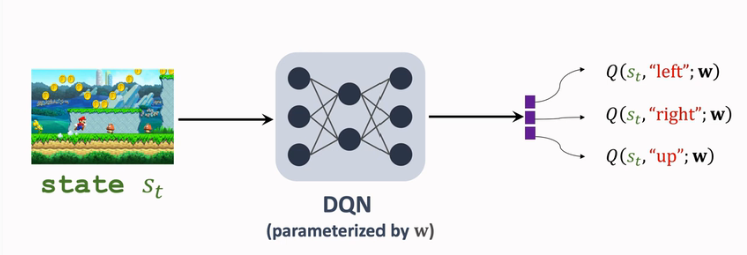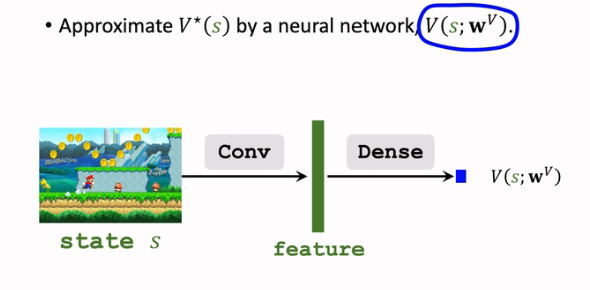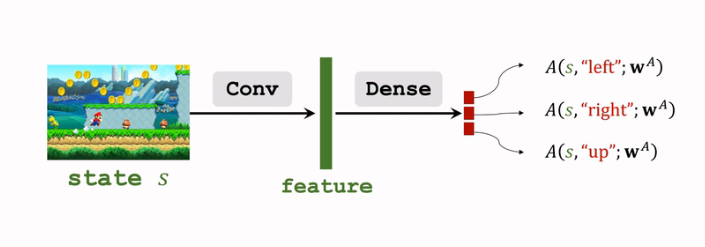# 强化学习-学习笔记12 | Dueling Network

Dueling Network 的应用范围不限于 DQN，本文只介绍其在 DQN上的应用。

## 12. Dueling Network

### 12.1 优势函数

1. 折扣回报：

$$U_t = R_t + \gamma \cdot R_{t+1} + \gamma^2R+...$$

2. 动作价值函数：

$$Q_\pi(s_t,a_t)=\mathbb{E}[U_t|S_t=s_t,A_t=a_t]$$

消去了未来的状态 和 动作，只依赖于当前动作和状态，以及策略函数 $$\pi$$

3. 状态价值函数：

$$V_\pi(s_t)=\mathbb{E}[Q_\pi(s_t,A)]$$

只跟策略函数 $$\pi$$ 和当前状态 $$s_t$$ 有关。

4. 最优动作价值函数

$$Q^*(s,a)=\mathop{max}\limits_{\pi}Q_\pi(s,a)$$

只依赖于 s,a，不依赖策略函数。

5. 最优状态价值函数

$$V^*(s)=\mathop{max}\limits_{a}V_\pi(S)$$

只依赖 S。

$$A^*(s,a)=Q^*(s,a)-V^*(s)$$

V* 作为 baseline ，优势函数的意思是动作 a 相对 V* 的优势，A*越好，那么优势就越大。

$$\mathop{max}\limits_{a}A^*(s,a)=0$$

Dueling Network 就是由定理二得到的。

### 12.2 Dueling Network 原理• 我们对于DQN的改进思路就是基于上面的定理2：$$Q^*(s,a)=V^*(s)+A^*(s,a)-\mathop{max}\limits_{a}A^*(s,a)$$

• 分别用神经网络 V 和 A 近似 V-star 和 A-star
• 即：$$Q(s,a;w^A,w^V)=V(s;w^V)+A(s,a;w^A)-\mathop{max}\limits_{a}A(s,a;w^A)$$
• 这样也完成了对于 Q-star 的近似，与 DQN 的功能相同。
• 首先需要用一个神经网络 $$V(s;w^V)$$ 来近似 $$V^*(s)$$• 用另一个神经网络$$A(s,a;w^A)$$$$A^*(s,a)$$ 进行近似:$Q(s,a;w)=V(s;w^V)+A(s,a;w^A)-\mathop{max}\limits_{a}A(s,a;w^A)$• 输入 状态 s，V 和 A 共享一些 卷积层，得到特征向量；
• 分别通过不同的全连接层，A输出向量，V输出实数；
• 通过上面的式子运算输出最终结果，是对所有动作的打分；

### 12.3 训练 Dueling Network

1. 经验回放 / 优先经验回放
2. Double DQN
3. M-step TD target

### 12.4 数学原理与不唯一性

• $$Q^*(s,a)=V^*(s)+A^*(s,a)$$
• $$Q^*(s,a)=V^*(s)+A^*(s,a)-\mathop{max}\limits_{a}A^*(s,a)$$

• 这是因为 等式1 有一个问题。

• 即我们无法通过学习 Q-star 来 唯一确定 V-star 和 A-star，即对于求得的 Q-star 值，可以分解成无数组 V-star 和 A-star。

• $$Q(s,a;w^A,w^V)=V(s;w^V)+A(s,a;w^A)-\mathop{max}\limits_{a}A(s,a;w^A)$$

• 我们是对 左侧Q 来训练整个 Dueling Network 的。如果 V 网络 向上波动 和 A 网络向下波动幅度相同，那么 Dueling Network 的输出完全相同，但是V-A两个网络都发生了波动，训练不好。

• 而加上最大化这一项就能避免不唯一性；即如果 V-star 向上波动10，A-star 向下波动10，那么整个式子的值会发生改变

因为max项随着A-star 的变化 也减少了10，总体上升了10

## x. 参考教程

posted @ 2022-07-09 13:58  climerecho  阅读(581)  评论(0编辑  收藏  举报